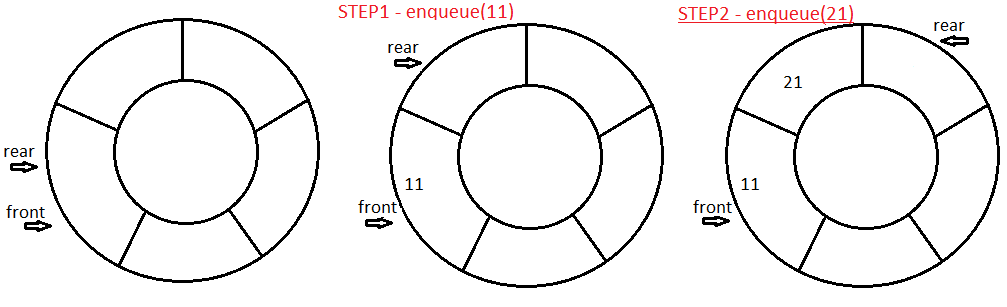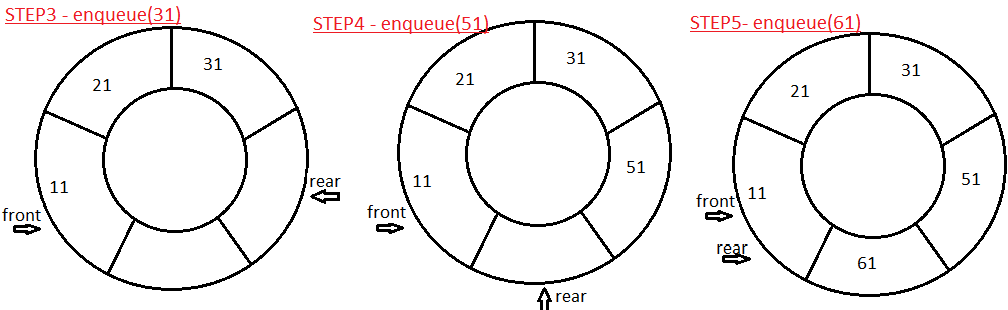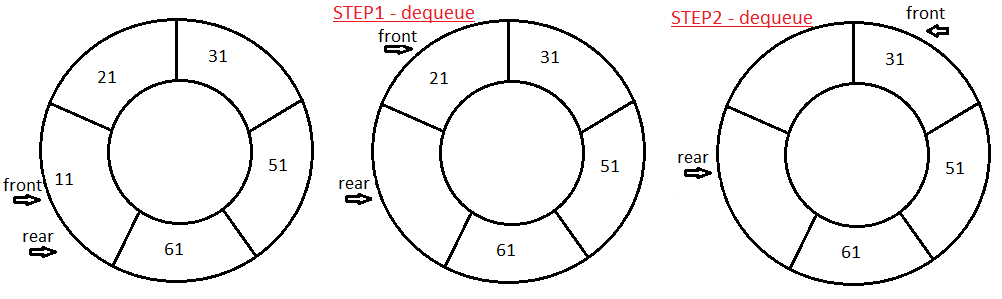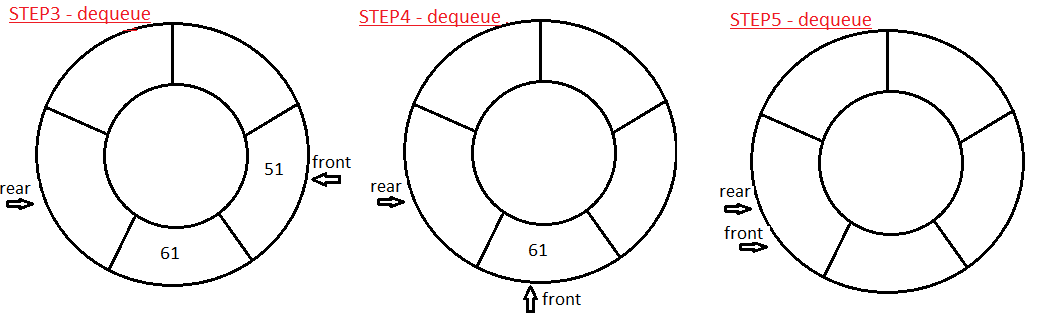### Circular Queue (with Generics) implementation in java

In this Data structures tutorial we will learn what is Circular Queues in java with example and program. We will learn how to implement your own Circular Queues with generics in java. We will learn how to insert and remove element from Circular Queues in java.

Circular Queue is Collection of entities or elements in which >
Addition of element (enqueue) is done at REAR.
Removal of element (dequeue) is done at FRONT.
Circular Queue follows FIFO (First in first out) - means  the first element added to the queue will be the first one to be removed.

Logic explanation(with Diagram) of Circular Queues in java >

I guess you  here you will find most comprehensive explanation of circular queue with diagram which will help you visualize how step by step we can enqueue and dequeue elements in circular queue.

Enqueue/Insert elements in Circular Queue>
Insert at rear at increment rear.Note:- No more elements can be added after completion of STEP5 as Circular Queue is full.

Dequeue\Remove elements from Circular Queue>
Delete from front and increment front.Note:- No more elements can be deleted after completion of STEP5 as Circular Queue is empty.

Full Program/Example of Circular Queues implementation with generics in java, insert and remove element from Circular Queues in java >
 class QueueFullException extends RuntimeException {       public QueueFullException(){        super();    }        public QueueFullException(String message){        super(message);    }     } class QueueEmptyException extends RuntimeException {    public QueueEmptyException(){        super();    }        public QueueEmptyException(String message){        super(message);    }     } /** * Circular Queue- implemented using Queue. */ class CircularQueue {       private E[] circularQueueAr;    private int maxSize;   //Maximum Size of Circular Queue       private int rear;//elements will be added/queued at rear.    private int front;   //elements will be removed/dequeued from front         private int number; //number of elements currently in Priority Queue         /**      * Constructor      */    public CircularQueue(int maxSize){        this.maxSize = maxSize;        circularQueueAr = (E[])new Object[this.maxSize];        number=0; //Initially number of elements in Circular Queue are 0.        front=0;        rear=0;       }    /**     * Adds element in Circular Queue(at rear)     */    public void enqueue(E item) throws QueueFullException {        if(isFull()){         throw new QueueFullException("Circular Queue is full");        }else{         circularQueueAr[rear] = item;         rear = (rear + 1) % circularQueueAr.length;            number++; // increase number of elements in Circular queue        }    }    /**     * Removes element from Circular Queue(from front)     */    public E dequeue() throws QueueEmptyException {        E deQueuedElement;        if(isEmpty()){         throw new QueueEmptyException("Circular Queue is empty");        }else{           deQueuedElement = circularQueueAr[front];         circularQueueAr[front] = null;         front = (front + 1) % circularQueueAr.length;            number--; // Reduce number of elements from Circular queue        }       return deQueuedElement;    }    /**     * Return true if Circular Queue is full.     */    public boolean isFull() {            return (number==circularQueueAr.length);       }    /**     * Return true if Circular Queue is empty.     */    public boolean isEmpty() {        return (number==0);    } } /** CircularQueueExample Main class - Copyright (c), AnkitMittal JavaMadeSoEasy.com */ public class CircularQueueExample {    public static void main(String[] args) {        CircularQueue circularQueue = new CircularQueue(5); //Initial size of CircularQueueArray             circularQueue.enqueue(11);        circularQueue.enqueue(21);        circularQueue.enqueue(31);        circularQueue.enqueue(51);        circularQueue.enqueue(61);        System.out.print("Elements deQueued from circular Queue: ");        System.out.print(circularQueue.dequeue()+" ");        System.out.print(circularQueue.dequeue()+" ");        System.out.print(circularQueue.dequeue()+" ");        System.out.print(circularQueue.dequeue()+" ");        System.out.print(circularQueue.dequeue()+" ");           }     } /*OUTPUT Elements deQueued from circular Queue: 11 21 31 51 61 */
If we analyze output of above Circular Queue program, we will find that- first element added to the circular queue was the first one to be removed in java.

Complexity of Circular Queues in java >

enqueue - O(1) [as we insert element at Rear in Circular Queue]
dequeue - O(1) [as we remove element from front in Circular Queue]

So in this Data structures tutorial we learned what is Circular Queues in java with example and program. We learned how to implement your own Circular Queues with generics in java. We learned how to insert and remove element from Circular Queues in java.

Having any doubt? or you you liked the tutorial! Please comment in below section.

1) Stacks, Queues in Data Structures in java

2) Single LinkedList implementations in Data Structures in java:-

3) Doubly LinkedList implementations in Data Structures in java:-

5) Some of the tricky and interesting Single LinkedList implementations in Data Structures in java

6) Binary tree tutorial in java >